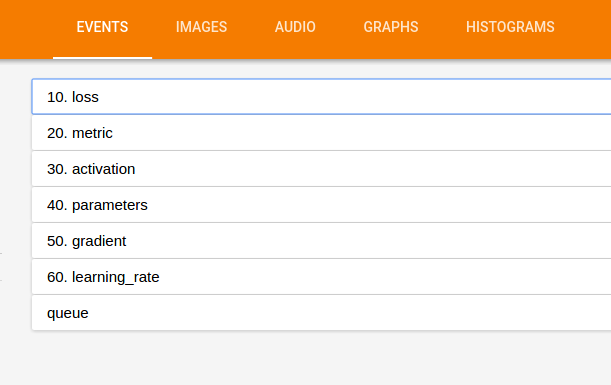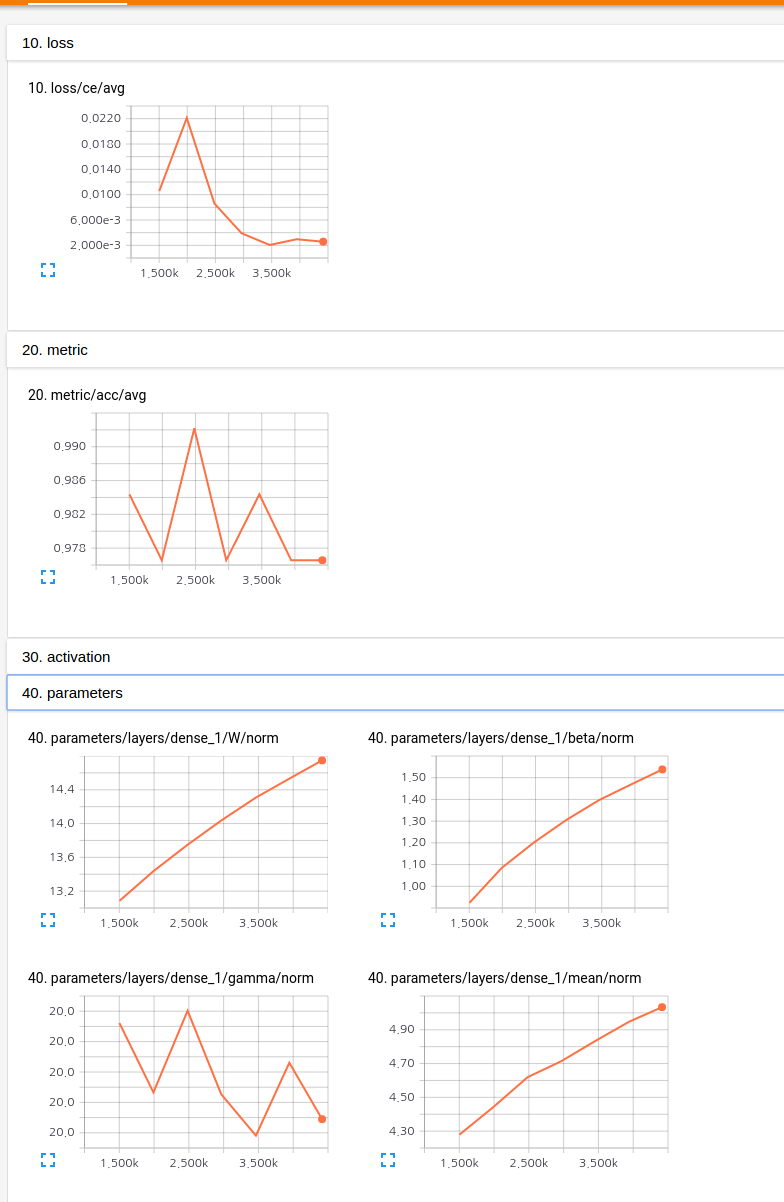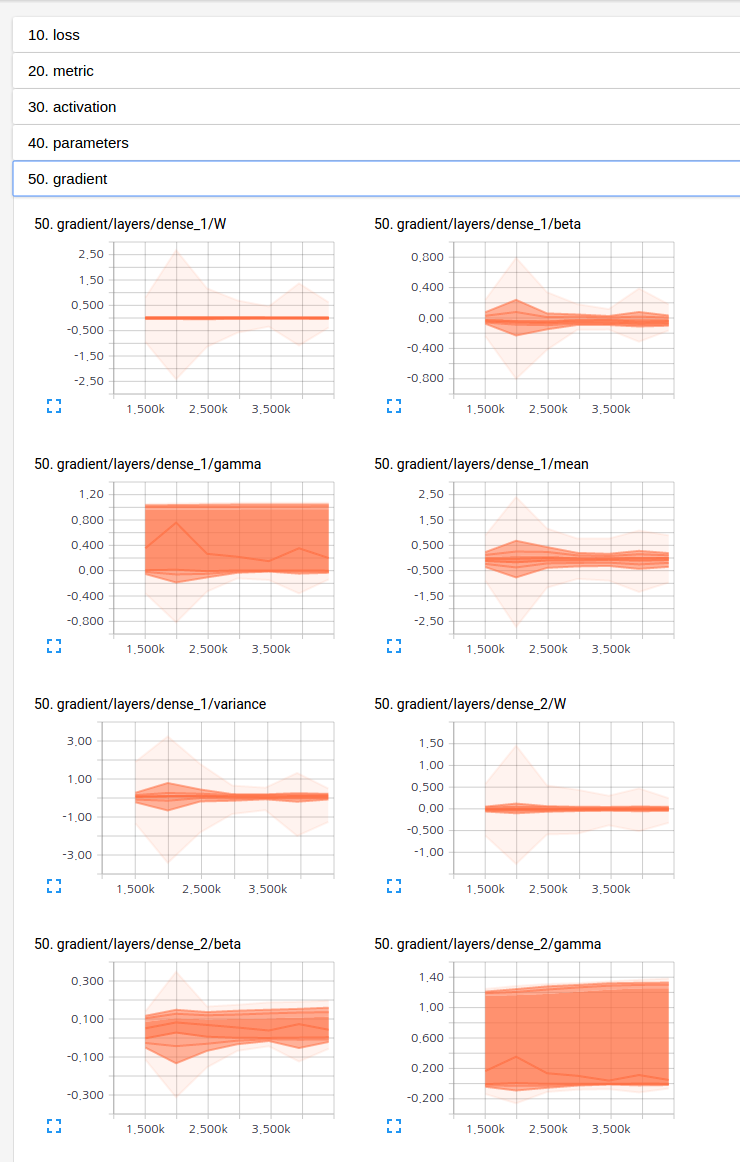Showing:

0

372

4yrs ago

6

0

MIT

# Sugar Tensor - A slim tensorflow wrapper that provides syntactic sugar for tensor variables

Sugar Tensor aims to help deep learning researchers/practitioners. It adds some syntactic sugar functions to tensorflow to avoid tedious repetitive tasks. Sugar Tensor was developed under the following principles:

Current Version : 1.0.0.2

## Principles

1. Don't mess up tensorflow. We provide no wrapping classes. Instead, we use a tensor itself so that developers can program freely as before with tensorflow.
2. Don't mess up the python style. We believe python source codes should look pretty and simple. Practical deep learning codes are very different from those of complex GUI programs. Do we really need inheritance and/or encapsulation in our deep learning code? Instead, we seek for simplicity and readability. For that, we use pure python functions only and avoid class style conventions.

## Installation

1. Requirements

1. tensorflow == 1.0.0
2. Dependencies ( Will be installed automatically )

1. tqdm >= 4.8.4
3. Installation

python 2

``pip install --upgrade sugartensor``

python 3

``pip3 install sugartensor``

docker installation : See docker README.md.

## Quick start

###Imports

``import sugartensor as tf   # no need of 'import tensorflow'``

## Features

### Sugar functions

All tensors--variables, operations, and constants--automatically have sugar functions which start with 'sg_' to avoid name space chaos. :-)

### Chainable object syntax

Inspired by prettytensor library, we support chainable object syntax for all sugar functions. This should improve productivity and readability. Look at the following snippet.

``````
logit = (tf.placeholder(tf.float32, shape=(BATCH_SIZE, DATA_SIZE))
.sg_dense(dim=400, act='relu', bn=True)
.sg_dense(dim=200, act='relu', bn=True)
.sg_dense(dim=10))
``````

### All returned objects are tensors.

In the above snippet, all values returned by sugar functions are pure tensorflow's tensor variables/constants. So, the following example is completely legal.

``````
ph = tf.placeholder(tf.float32, shape=(BATCH_SIZE, DATA_SIZE)   # <-- this is a tensor
ph = ph.sg_dense(dim=400, act='relu', bn=True)   # <-- this is a tensor
ph = ph * 100 + 10  # <-- this is ok.
ph = tf.reshape(ph, (-1, 20, 20, 1)).conv(dim=30)   # <-- all tensorflow's function can be applied and chained.
``````

### Practical DRY (Don't repeat yourself) functions for deep learning researchers

We provide pre-defined powerful training and report functions for practical developers. The following code is a full mnist training module with saver, report and early stopping support.

``````
# -*- coding: utf-8 -*-
import sugartensor as tf

# MNIST input tensor ( with QueueRunner )
data = tf.sg_data.Mnist()

# inputs
x = data.train.image
y = data.train.label

# create training graph
logit = (x.sg_flatten()
.sg_dense(dim=400, act='relu', bn=True)
.sg_dense(dim=200, act='relu', bn=True)
.sg_dense(dim=10))

# cross entropy loss with logit ( for training set )
loss = logit.sg_ce(target=y)

# accuracy evaluation ( for validation set )
acc = (logit.sg_reuse(input=data.valid.image).sg_softmax()
.sg_accuracy(target=data.valid.label, name='val'))

# train
tf.sg_train(loss=loss, eval_metric=[acc], ep_size=data.train.num_batch)
``````

You can check all statistics through the tensorboard's web interface like the following.If you want to write another more complex training module without repeating saver, report, or whatever, you can do that like the following.

``````
# def alternate training func
@tf.sg_train_func   # <-- sugar annotator for training function wrapping
def alt_train(sess, opt):
l_disc = sess.run([loss_disc, train_disc])  # training discriminator
l_gen = sess.run([loss_gen, train_gen])  # training generator
return np.mean(l_disc) + np.mean(l_gen)

# do training
alt_train(log_interval=10, ep_size=data.train.num_batch, early_stop=False, save_dir='asset/train/gan')
``````

Please see the example codes in the 'sugartensor/example/' directory.

### Custom layers

You can add your own custom layer functions like the following code snippet.

``````# residual block
@tf.sg_sugar_func
def sg_res_block(tensor, opt):
# default rate
opt += tf.sg_opt(size=3, rate=1, causal=False)

# input dimension
in_dim = tensor.get_shape().as_list()[-1]

# reduce dimension
input_ = (tensor
.sg_bypass(act='relu', bn=(not opt.causal), ln=opt.causal)
.sg_conv1d(size=1, dim=in_dim/2, act='relu', bn=(not opt.causal), ln=opt.causal))

# 1xk conv dilated
out = input_.sg_aconv1d(size=opt.size, rate=opt.rate, causal=opt.causal, act='relu', bn=(not opt.causal), ln=opt.causal)

# dimension recover and residual connection
out = out.sg_conv1d(size=1, dim=in_dim) + tensor

return out

# inject residual block
tf.sg_inject_func(sg_res_block)
``````

### Multip GPU support

You can train your model with multiple GPUs using sg_parallel decorator as follow:

``````# batch size
batch_size = 128

# MNIST input tensor ( batch size should be adjusted for multiple GPUS )
data = tf.sg_data.Mnist(batch_size=batch_size * tf.sg_gpus())

# split inputs for each GPU tower
inputs = tf.split(data.train.image, tf.sg_gpus(), axis=0)
labels = tf.split(data.train.label, tf.sg_gpus(), axis=0)

# simple wrapping function with decorator for parallel training
@tf.sg_parallel
def get_loss(opt):

# conv layers
with tf.sg_context(name='convs', act='relu', bn=True):
conv = (opt.input[opt.gpu_index]
.sg_conv(dim=16, name='conv1')
.sg_pool()
.sg_conv(dim=32, name='conv2')
.sg_pool()
.sg_conv(dim=32, name='conv3')
.sg_pool())

# fc layers
with tf.sg_context(name='fcs', act='relu', bn=True):
logit = (conv
.sg_flatten()
.sg_dense(dim=256, name='fc1')
.sg_dense(dim=10, act='linear', bn=False, name='fc2'))

# cross entropy loss with logit
return logit.sg_ce(target=opt.target[opt.gpu_index])

# parallel training ( same as single GPU training )
tf.sg_train(loss=get_loss(input=inputs, target=labels), ep_size=data.train.num_batch)

``````

# Author

Namju Kim (namju.kim@kakaobrain.com) at KakaoBrain Corp.

## Rate & Review

Great Documentation0
Easy to Use0
Performant0
Highly Customizable0
Bleeding Edge0
Responsive Maintainers0
Poor Documentation0
Hard to Use0
Slow0
Buggy0
Abandoned0
Unwelcoming Community0
100